Enable Dark Mode!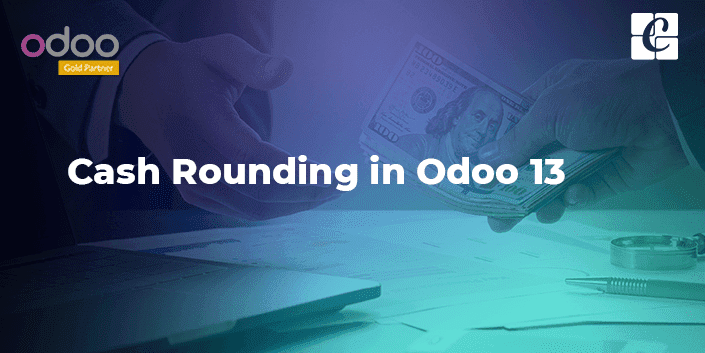By: Shabna P

# Cash Rounding in Odoo 13

## Functional Odoo 13 Accounting

Cash rounding in a business process involves changing the denomination to more exact value that can be made easy to pay. Sometimes circulations of some coins are taken out or those of payment become impractical to exact. Also, there may be a voluntary practice to round to a fixed value as there persists lack of cash denominations.

Odoo supports such kind of cash rounding methods to a higher value or to a lower value or to half up value, for the total amount of a bill to the nearest 5 cents. This helps the vendor to create a more close and rounded figured bill.

To enable rounding function, Go To Accounting module -> Configuration -> Settings -> Taxes -> Rounding method.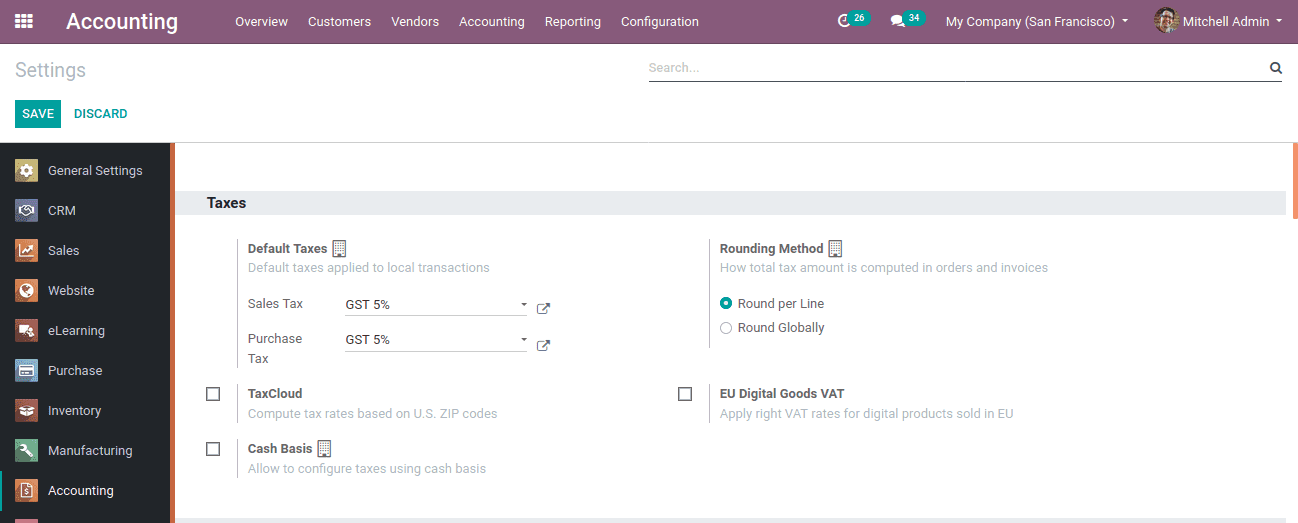Rounding method defines how the total amount is computed in orders and invoices. There are two methods:

1. Round per line: Used when the price is tax included, that way it adjusts the sum of the line of subtotals equaling the total with taxes.

2. Round Globally: Used when the tax is included but it adjusts on the total tax amount.

Now create cash rounding through, Configuration -> Management -> Cash Rounding and CREATE.

The parameters in the cash rounding window include:

Name:  Here one can specify the name of Rounding

Rounding Precision: Precision means the numeric value describing the number of digits expressing the particular value both left and right of the decimal. Hence rounding precision will affect the computed value.

Rounding strategy: This can be of two types. Add round a line and modify the tax amount. In add round, a line strategy, add the rounding in a new line while modifying tax amount strategy, add the rounding to the highest amount of tax.

Account: It defines the account where the rounding will go.

Rounding Method: It defines to which precise value it is rounding, either UP, DOWN or HALF-UP.UP:  It round to higher infinity depending on the rounding precision

DOWN: It round to lower affinity depending on the rounding precision

HALF-UP:  When the fraction part is equal to or greater than 0.5 then round to higher infinity or if less than 0.5 then round to lower infinity.

Then SAVE. Thus a cash rounding is created.

Now let's look at how to Add a rounding line works.

Different cash rounding methods can be applied to the invoice. For that create an invoice for a customer, Accounting Module -> Customer -> Invoice -> Create.

Create an invoice by adding customers and products, where the product amount is a decimal value. In other info tab add cash rounding method as ‘rounding up’.Now the changes can be seen at the invoice as the total amount changes to higher infinity value. Thus the amount 752.56 can be changed to 752.60.If the cash rounding method is DOWN, Let’s look at how the total amount changes.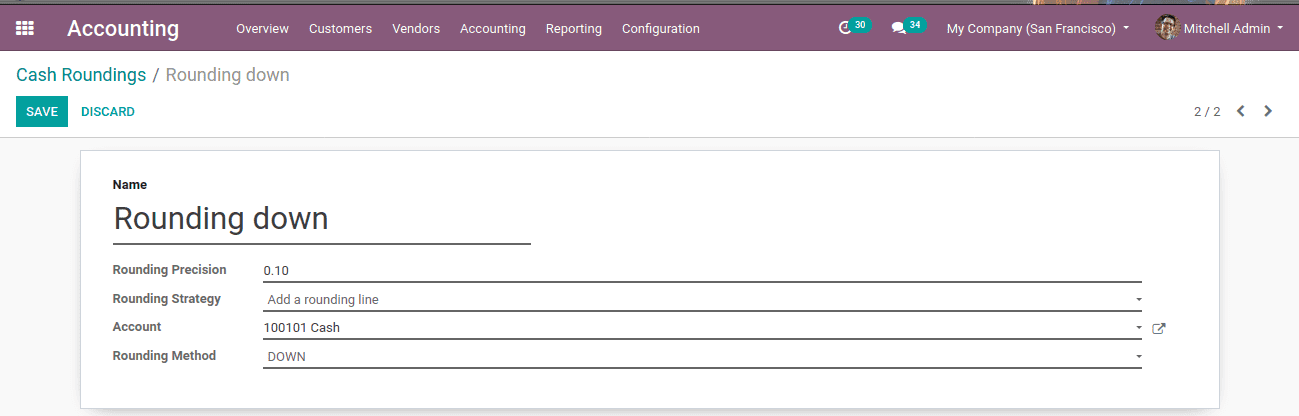Create an invoice as above, insert customer and product whose price is a decimal value. Also in other info tab, add cash rounding method as ‘rounding down’.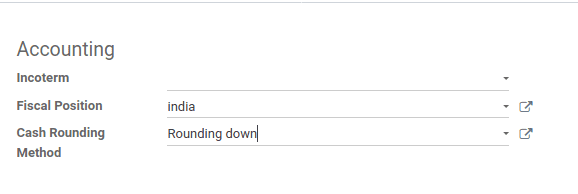Now look at the invoice and see the changes. Amount 752.56 rounded to 752.50.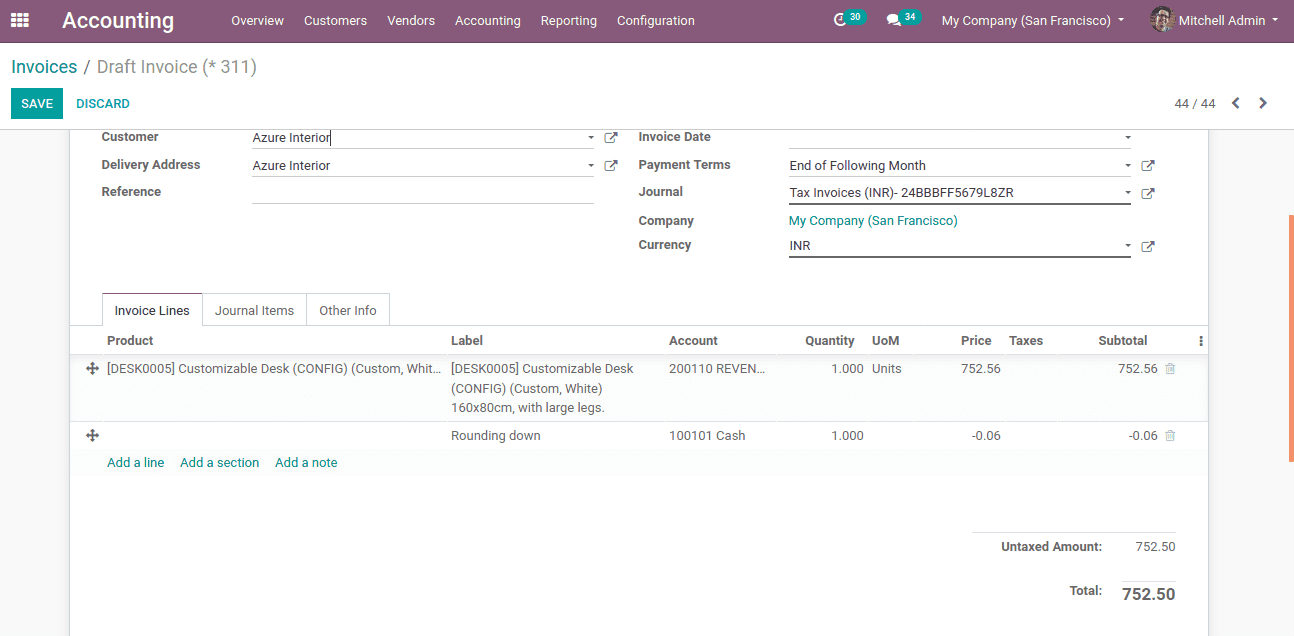Now the cash rounding method is HALF-UP. Let's look at how the total amount changes.Create an invoice as above, insert customer and product whose price is a decimal value. Also in other info tab add cash rounding method as ‘rounding half-up’.Now look at the invoice and see the changes. Amount 752.45 is rounded to 752.50.2. Modify tax amount

Next case of Modify tax amount, cash rounding given as UP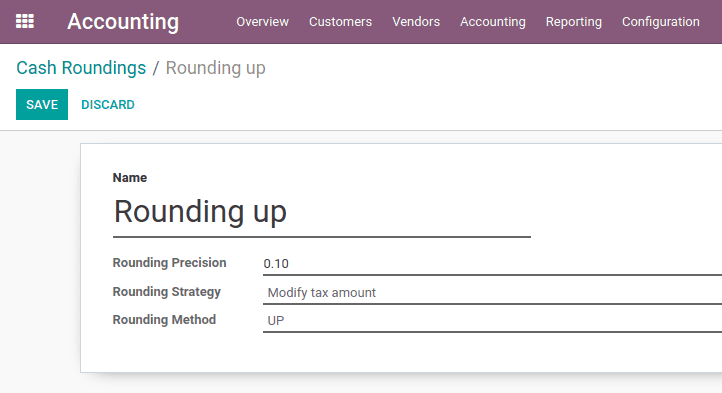Create an invoice as above, insert customer and product whose price is a decimal value. Here tax amount is 40.02 and modifying the tax amount strategy, the tax amount will be adjusted.Also in other info tab, add cash rounding method as ‘rounding up’.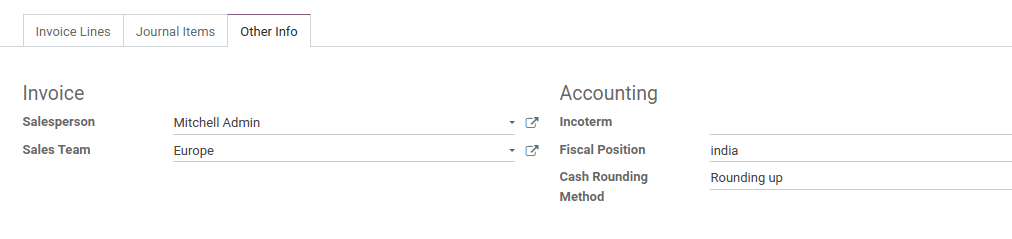Here the tax amount adjusted to 40.10.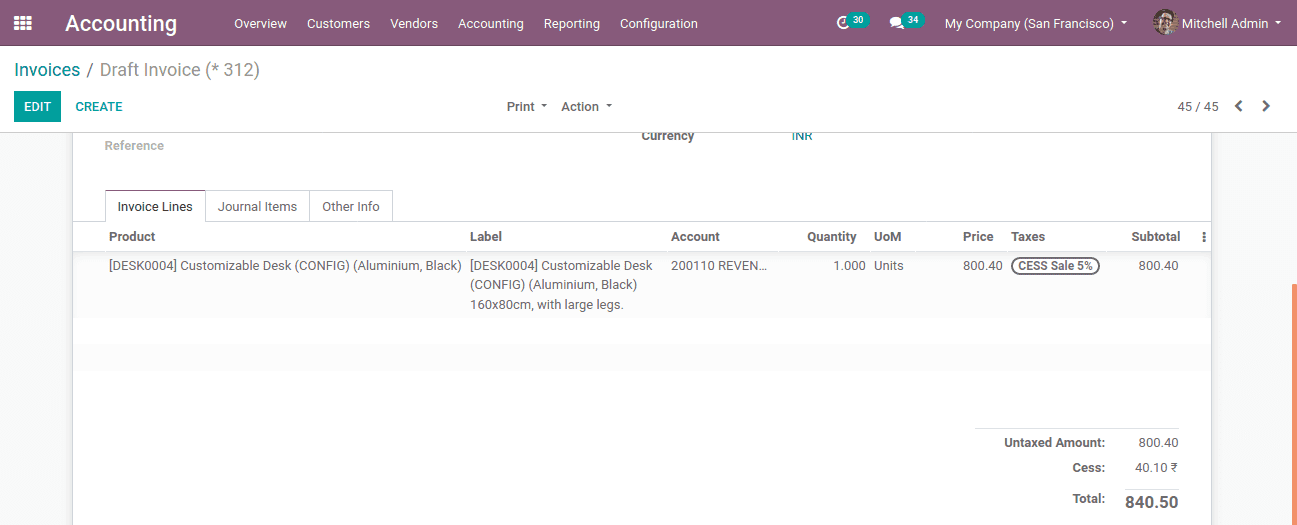Now another case of cash rounding given as DOWN.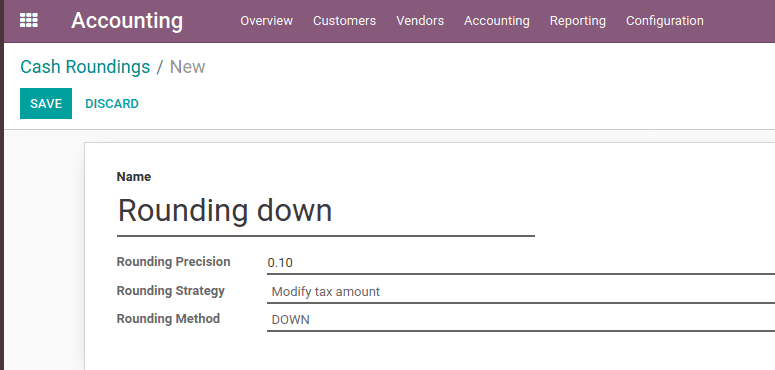Create an invoice as above, insert customer and product whose price is a decimal value.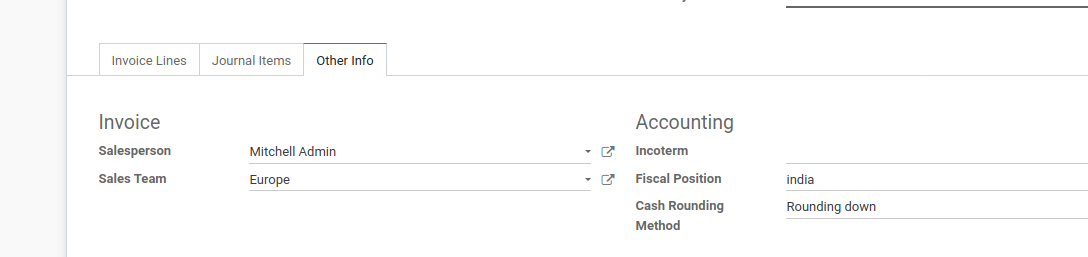Also in other info, tab add cash rounding method as ‘rounding down’. Here the tax amount is adjusted to 40.00.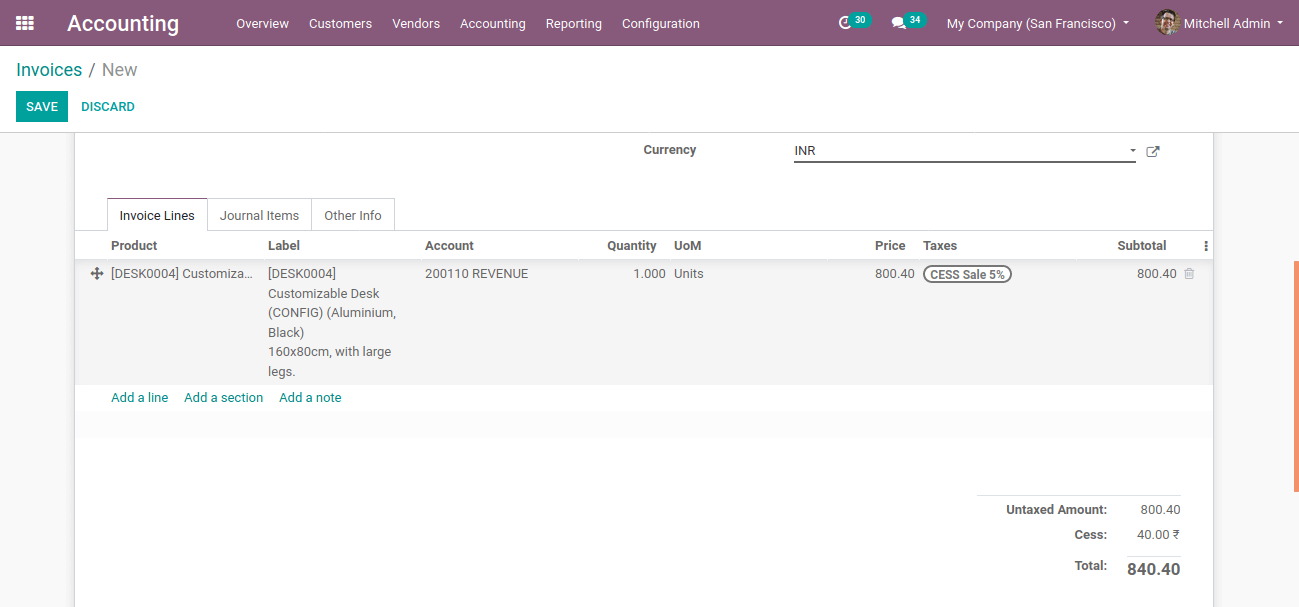Next case of cash rounding is given as HALF-UP.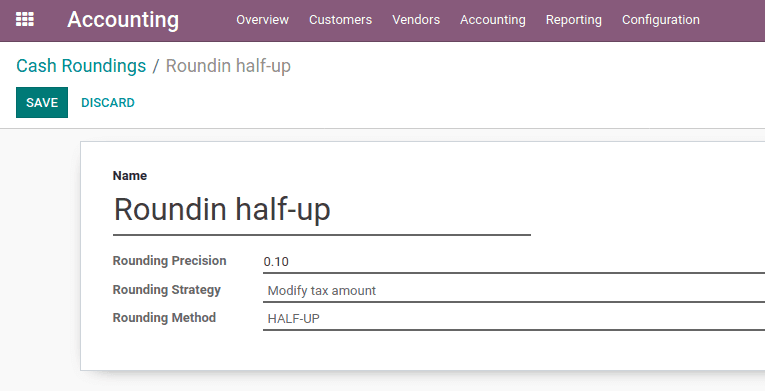Create an invoice as above, insert customer and product whose price is a decimal value.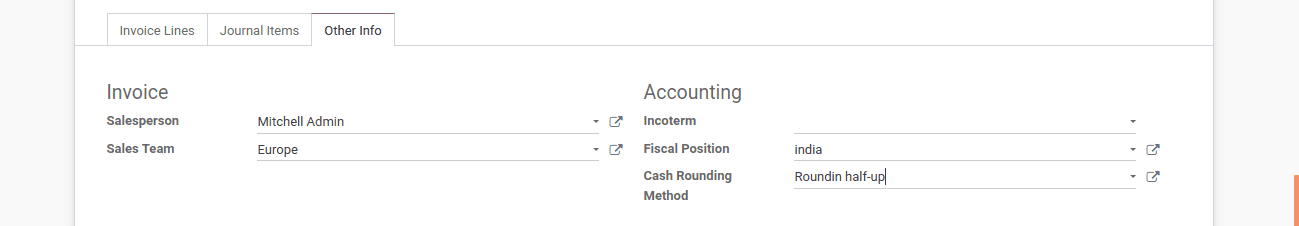Also in other info tab add cash rounding method as ‘rounding down’.Here also the total tax amount adjusted to 40.09. This is how cash rounding works in Odoo.

If you need any assistance in odoo, we are online, please chat with us.

### Recent Posts

#### How to Plan a Task in Odoo 16 Field Services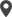Calicut

Cybrosys Technologies Pvt. Ltd.
Neospace, Kinfra Techno Park
Kakkancherry, Calicut
Kerala, India - 673635London

Cybrosys Limited
Alpha House,
100 Borough High Street, London,
SE1 1LB, United KingdomKochi

Cybrosys Technologies Pvt. Ltd.
1st Floor, Thapasya Building,
Kochi, India - 682030.Bangalore

Cybrosys Techno Solutions
The Estate, 8th Floor,